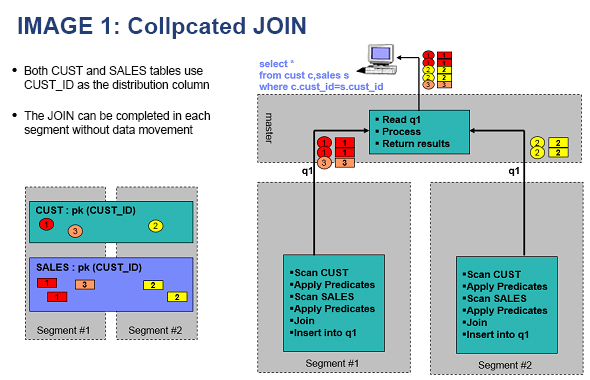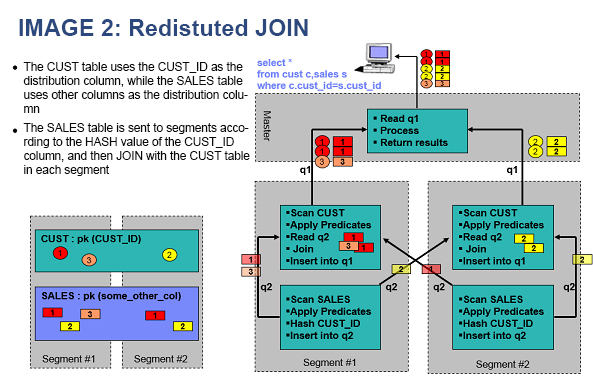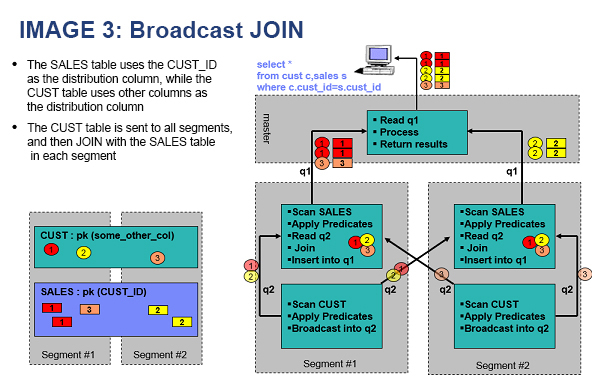## Table distribution options

AnalyticDB for PostgreSQL provides three options to distribute the data of a table across compute nodes: hash distribution, random distribution, and replicated distribution.
``CREATE TABLE table_name (...) [ DISTRIBUTED BY (column  [,..] ) | DISTRIBUTED RANDOMLY | DISTRIBUTED REPLICATED ]``
Note AnalyticDB for PostgreSQL V4.3 only supports hash distribution and random distribution. Replicated distribution is a new feature in AnalyticDB for PostgreSQL V6.0.
The `CREATE TABLE` statement supports the following clauses that specify table distribution options:
• `DISTRIBUTED BY (column, [ ... ])`: specifies hash distribution. The rows of the table are distributed across compute nodes based on their hash values in the distribution column selected as the distribution key. Each row is assigned to one compute node. Rows with identical values are always assigned to the same compute node. You can choose a unique distribution key (for example, the primary key of the table) to ensure even distribution of data. The default table distribution option is hash distribution. If you do not specify a DISTRIBUTED clause, the table uses its primary key or the first identified suitable distribution column as the distribution key. If no suitable distribution column is identified, the system uses random distribution.
• `DISTRIBUTED RANDOMLY`: specifies random distribution. The rows of the table are evenly distributed across all compute nodes by using a round-robin algorithm. Rows with identical values may be assigned to different compute nodes. We recommend that you only use random distribution when no suitable distribution column is identified.
• `DISTRIBUTED REPLICATED`: specifies replicated distribution. All data of the table is stored on all compute nodes. This means that each compute node stores the same rows. If you want to join large and small tables, you can specify replicated distribution for small tables to increase join performance.Examples:

In the following example, a table that uses hash distribution is created. Each row is assigned to one compute node based on its hash value.
``````CREATE TABLE products (name varchar(40),
prod_id integer,
supplier_id integer)
DISTRIBUTED BY (prod_id);                ``````
In the following example, a table that uses random distribution is created. The rows of the table are distributed across all compute nodes by using a round-robin algorithm. If no suitable distribution column is identified, we recommend that you use random distribution.
``````CREATE TABLE random_stuff (things text,
etc text)
DISTRIBUTED RANDOMLY;``````
In the following example, a table that uses replicated distribution is created. All data of the table is stored on all compute nodes.
``````CREATE TABLE replicated_stuff (things text,
etc text)
DISTRIBUTED REPLICATED;``````
For simple queries that use a distribution key, AnalyticDB for PostgreSQL filters compute nodes based on the distribution key before sending query requests to them. Such simple queries include those initiated by UPDATE and DELETE statements. For example, if you query data from the products table that uses prod_id as the distribution key, your query is only sent to the compute nodes whose values of prod_id are 101. This increases your query performance.
``select * from products where prod_id = 101;``

## Hash keys

To increase query performance, we recommend that you choose a distribution column as the distribution key for a table based on the following rules:

• Choose one or more distribution columns with data distributed evenly. If the distribution columns you choose have unevenly distributed data, they may skew the data in the table. Tables with skewed data have one or more compute nodes with a disproportionate number of rows. In this situation, some compute nodes finish their portion of a parallel query before the others. However, based on the Cannikin Law, the query cannot be completed until all compute nodes finish processing. As a result, the query is only as fast as the slowest compute node. Therefore, we recommend that you do not choose distribution columns with Boolean or date values.
• Choose a distribution column that is frequently used in JOIN clauses. This way, you can join two tables by using a collocated join, as shown in the following figures. If the join key is the same as the distribution key, the join can be completed within the associated compute nodes without data movement. If you do not choose a distribution column that is frequently used in JOIN clauses, you must redistribute (redistribute motion) the larger one of the two tables you want to join and then perform a redistributed join. You also have the option to broadcast (broadcast motion) the smaller one of the two tables and then perform a broadcast join. Both the redistribute and broadcast motions increase network overheads.
• Choose a frequently used query criterion as the distribution key. This enables AnalyticDB for PostgreSQL to filter compute nodes based on the distribution key before it sends query requests to them.
• If you do not specify a distribution key, the primary key of the table is used as the distribution key. In addition, if the table does not have a primary key, the first column is used as the distribution key.
• The distribution key can be defined from one or more columns. Example:
``create table t1(c1 int, c2 int) distributed by (c1,c2);``
• Exercise caution when you choose random distribution because it does not support collocated joins or compute node filtering.## Limits on distribution keys

• A column defined as the distribution key of a table cannot be updated.
• The distribution key of a table must be either the primary key or a unique key. Example:
``create table t1(c1 int, c2 int, primary key (c1)) distributed by (c2);``
Note In this example, the primary key c1 differs from the distribution key c2. As a result, the execution of the statement fails and the system reports the following error:
``ERROR: PRIMARY KEY and DISTRIBUTED BY definitions incompatible``
• A column with Geometry values or any other custom data type cannot be used as the distribution key of a table.

## Troubleshooting for data skew

If the query performance of a table is poor, check whether an inappropriate distribution key is specified. Example:
``create table t1(c1 int, c2 int) distributed by (c1);``
For this example, execute the following statement to check for data skew in the table:
``````select gp_segment_id,count(1) from  t1 group by 1 order by 2 desc;
gp_segment_id | count
---------------+--------
2 | 131191
0 |     72
1 |     68
(3 rows)``````
If you find that some compute nodes store more rows than the others, data skew occurs. We recommend that you define a column with evenly distributed data as the distribution column. For the following example, execute the `ALTER TABLE` statement to specify the c2 column as the distribution column:
``alter table t1 set distributed by (c2);``

The distribution key of the t1 table is changed to the c2 column. After the t1 table is redistributed based on the c2 column, its data is no longer skewed.